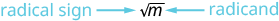## Key Concepts

• Square Root Notation $\sqrt{m}$ is read ‘the square root of $m$ ’.  If $m={n}^{2}$ , then $\sqrt{m}=n$ , for $n\ge 0$ .• Use a strategy for applications with square roots.
• Identify what you are asked to find.
• Write a phrase that gives the information to find it.
• Translate the phrase to an expression.
• Simplify the expression.
• Write a complete sentence that answers the question.